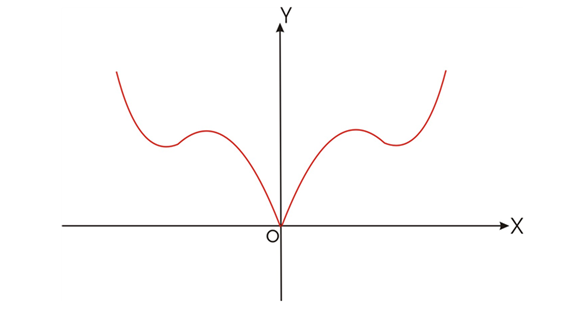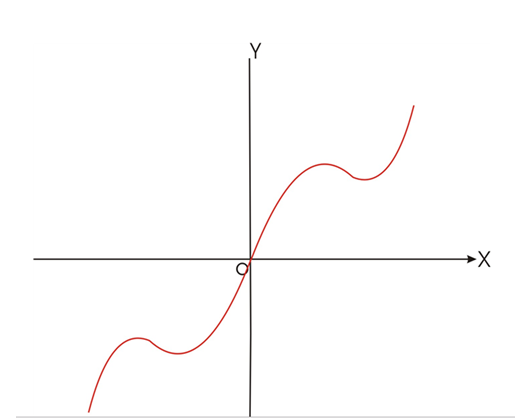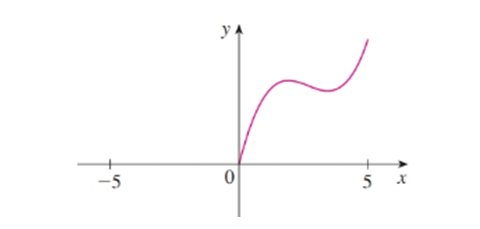# Plot the graph.### Single Variable Calculus: Concepts...

4th Edition
James Stewart
Publisher: Cengage Learning
ISBN: 9781337687805### Single Variable Calculus: Concepts...

4th Edition
James Stewart
Publisher: Cengage Learning
ISBN: 9781337687805

#### Solutions

Chapter 1.1, Problem 66E

a.

To determine

## Plot the graph.

Expert Solution### Explanation of Solution

Given information:

A function f has domain [5,5] and a portion of its graph is shown.

Complete the graph of f if it is known that f is even.Calculation:

If f is an even function the completed graph will be symmetric about Y-axis

Hence the graph required isb.

To determine

### Plot the graph.

Expert Solution### Explanation of Solution

Given information:

A function f has domain [5,5] and a portion of its graph is shown.

Complete the graph of f if it is known that f is odd.Calculation:

If f is an odd function, the completed graph will be symmetric about the origin. Hence the required graph is### Have a homework question?

Subscribe to bartleby learn! Ask subject matter experts 30 homework questions each month. Plus, you’ll have access to millions of step-by-step textbook answers!